# De la Vallée-Poussin sum

The expression(*)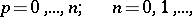where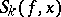,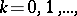are the partial sums of the Fourier series of a functionwith period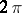. If, the de la Vallée-Poussin sums become identical with the partial Fourier sums, and if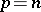, they become identical with the Fejér sums (cf. Fejér sum). Ch.J. de la Vallée-Poussin ,  was the first to study the method of approximating periodic functions by polynomials of the form (*); he also established the inequality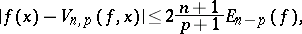whereis the best uniform approximation of the functionusing trigonometric polynomials of order not greater than. If,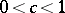and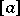is the integer part of the number, the polynomials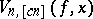realize an approximation of order. The polynomialsyield the best order approximations of continuous functions of period, with an estimate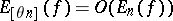for certain values of,. The de la Vallée-Poussin sums have several properties which are of interest in the theory of summation of Fourier series. For instance, if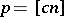,, then, and ifis a trigonometric polynomial of order not exceeding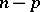, then. A de la Vallée-Poussin sum may be written as follows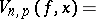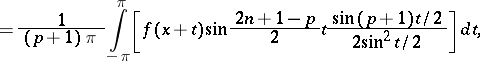where the expressions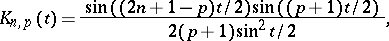are said to be the de la Vallée-Poussin kernels.